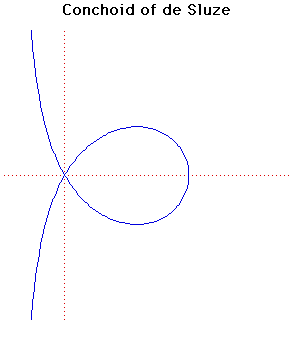# Curves

### Conchoid of de SluzeCartesian equation:
$a(x + a)(x^{2} + y^{2}) = k^{2}x^{2}$
Polar equation:
$a(r \cos( \theta ) + a) = k^{2}\cos^{2}( \theta )$

### Description

This curve was first constructed by René de Sluze in 1662.

René Francois Walter- Baron de Sluze was an important man in the church as well as a mathematician. He contributed to the geometry of spirals and the finding of geometric means. He also invented a general method for determining points of inflection of a curve.

### Associated Curves

Definitions of the Associated curves